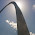## Thursday, April 9, 2015

### Playing with mesh curvature in real-time

Boomer labs was show casing a cool new map plugin for 3dsMax that uses curvature.
Check it out here: https://vimeo.com/124072533

So I got inspired to play around with curvature in a real-time shader.

I knew about ddx and ddy and then found my way to a cool webGL shader by Evan Wallace.
``http://madebyevan.com/shaders/curvature/``

Plugging this into ShaderFX inside 3dsMax and a few tweaks later we get:

``````
``````
``I used a custom code node inside ShaderFX, so below is the code I ended up using.``
``````
``````
``I noticed that the curvature colors flip around on the other side of the world axis (so the back side of the model). I didn't have the time to figure out why that is yet.``
``````

float ComputeCurvature( float3 vertex, float3 normal, float strength)
{
float3 n = normalize(normal);

float3 dx = ddx(n);
float3 dy = ddy(n);
float3 xneg = n - dx;
float3 xpos = n + dx;
float3 yneg = n - dy;
float3 ypos = n + dy;
float depth = length(vertex);
float curvature = (cross(xneg, xpos).y - cross(yneg, ypos).x) * strength / depth;

return curvature;
}

struct CurvatureCalcOutput
{
float curvature;
float negative;
float positive;
float3 color;
};

CurvatureCalcOutput CurvatureCalcFunc(float3 vertex_pos, float3 normal, float strength)
{
CurvatureCalcOutput OUT;

OUT.curvature = ComputeCurvature(vertex_pos, normal, strength);
OUT.positive = clamp(-OUT.curvature, 0, 1);
OUT.negative = clamp(OUT.curvature, 0, 1);

OUT.color = float3(0,1,0);

OUT.color = lerp( OUT.color, float3(1,0,0), OUT.negative);
OUT.color = lerp( OUT.color, float3(0,0,1), OUT.positive);

return OUT;
}
``````
``````
``````

1.Did you ever figure out the world axis flip thing?

2.Could this work in Maya?

3.Works just fine in Maya

4.This comment has been removed by the author.